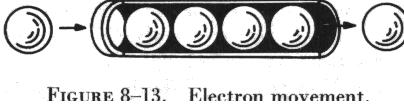CURRENTFLOW CURRENT FLOW

Electrons in motion make up an electric current. This electric current is usually referred to as "current" or "current flow," no matter how many electrons are moving. When the current flow is in one direction only, it is called direct current. Later in the study of electrical fundamentals, current that reverses itself periodically, called alternating current, will be discussed. In the present study all references are to direct current.

Since an electric current may consist of varying numbers of electrons, it is important to know the number of electrons flowing in a circuit in a given time. Electrons can be counted by measuring the basic electrical charge on each electron.

Since this charge is very small, a practical unit, the coulomb, is used to measure an amount, or quantity, of electrical charge. The accumulated charge on 6.28 billion billion electrons is called one coulomb. When this quantity of electrons flows past a given point in an electrical circuit, one ampere of current is said to be flowing in the circuit. Current flow is measured in amperes or parts of amperes by an electrical instrument called an ammeter. The symbol used to indicate current in formulas or on schematics is the capital letter "I," which stands for the intensity of current flow.The drift of free electrons must not be confused with the concept of current flow that approaches the speed of light. When a voltage is applied to a circuit, the free electrons travel but a short distance before colliding with atoms. These collisions usually knock other electrons free from their atoms, and these electrons travel on toward the positive terminal of the wire, colliding with other atoms as they drift at a comparatively slow rate of speed. To understand the almost instantaneous speed of the effect of electric current, it is helpful to visualize a long tube filled with steel balls as shown in figure 8-13.

It can be seen that a ball introduced in one end of the tube, which represents a conductor, will immediately cause a ball to be emitted at the opposite end of the tube. Even if the tube were long enough to reach clear across the country, this effect could still be visualized as being instantaneous. Thus, electric current flow can be viewed as occurring instantaneously, even though it is a result of a comparatively slow drift of electrons.